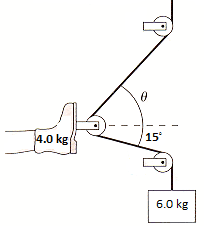# Traction and a Broken Leg

In summary, the conversation involves finding the tension in a rope and the proper angle for the upper rope in a traction setup for an accident victim with a broken leg. By using Newton's laws and the equation w=mg, the tension in the rope is calculated to be 58.8 N. To find the proper angle for the upper rope, the equation 58.8 sin(θ) = 39.2 + 58.8 sin(15°) is used, resulting in an angle of 67.74°. The net traction force pulling on the leg is then calculated to be 79 N. The person seeking help had some difficulty understanding the steps, but with clarification, they were able to solve the problem correctly

## Homework Statement

An accident victim with a broken leg is being placed in traction. The patient wears a special boot with a pulley attached to the sole. The foot and boot together have a mass of m = 4.0 kg, and the doctor has decided to hang a 6.0 kg mass from the rope. The boot is held suspended by the ropes, as shown in the image, and does not touch the bed.a) Determine the amount of tension in the rope by using Newton's laws to analyze the hanging mass.
b) The net traction force needs to pull straight out on the leg. What is the proper angle θ for the upper rope?
c) What is the net traction force pulling on the leg?

--------------
I have seen other people post this problem with different circumstances/variables, however, when I plug in my variables and try to make it work for my given problem I am finding myself stuck.

w=mg

## The Attempt at a Solution

a)[/B] Find the tension on the rope:
w = mg
= (6.0 kg)(9.8 [gravity])
w = 58.8 N (rounded to 59N) tension on the rope

b) Find the proper angle for the upper rope:
First I try to find the amount of force on the boot. using the same equation
w = mg
= (4.0kg)(9.8)
= 39.2 N of force acting on the boot
Then I take the sine to find the correct angle, right? So,
58.8 sin(θ) = 39.2 + 58.8 sin(15°)
θ = 54.42°
But I know that is incorrect. My book says that it's actually 67.8°. How? What am I doing wrong?

c) I can't even get to this point without answering (b).

b) Find the proper angle for the upper rope:
First I try to find the amount of force on the boot. using the same equation
w = mg
= (4.0kg)(9.8)
= 39.2 N of force acting on the boot
Then I take the sine to find the correct angle, right? So,
58.8 sin(θ) = 39.2 + 58.8 sin(15°)
θ = 54.42°
58.8sin(θ) = 54.42°
sin(θ) = 54.42 / 58.8
sin(θ) = 0.9255
sin-1(0.9255) = 67.74°

That is close to 67.8°...but I am not sure if I did it correctly or not.

From there:
c) Tcos(15°)+Tcos(67.7°) = 1.345382
= (1.345382)(58.8°)
= 79.12N (rounded to 79N) net traction force pulling on the leg

I am shooting in the dark here and am not sure if I am even doing it correctly. Thanks in advance

θ = 54.42°
I think you mean 58.8 sin(θ)=54.42.
You answer in part c looks ok, but you are a bit careless in writing out your steps. The net force is 1.345382T, not just 1.345382, and the 58.8 is Newtons, not degrees.

b) Find the proper angle for the upper rope:
First I try to find the amount of force on the boot. using the same equation
w = mg
= (4.0kg)(9.8)
= 39.2 N of force acting on the boot
Then I take the sine to find the correct angle, right? So,
58.8 sin(θ) = 39.2 + 58.8 sin(15°)
θ = 54.42°
58.8sin(θ) = 54.42°
sin(θ) = 54.42 / 58.8
sin(θ) = 0.9255
sin-1(0.9255) = 67.74°

That is close to 67.8°...but I am not sure if I did it correctly or not.

From there:
c) Tcos(15°)+Tcos(67.7°) = 1.345382
= (1.345382)(58.8°)
= 79.12N (rounded to 79N) net traction force pulling on the leg

I am shooting in the dark here and am not sure if I am even doing it correctly. Thanks in advance

You did indeed do it right, and you helped me much. Although it was difficult to decypher exactly what you were doing. Your algebra wasnt clear. Thank you alot. You helped me much.